Intelligent Robot Lab
Brown University, Providence RI

# The Fourier Basis

The Fourier basis is a simple, principled basis function scheme for linear value function approximation in reinforcement learning. It has performed well over a wide range of problems, despite its simplicity, and I now use it almost exclusively. My advice to fellow researchers who require function approximation is that the Fourier basis should be the first thing you try.

If a problem is small enough, the Fourier basis avoids the need for extensive problem-specific feature engineering. For example, we have achieved good results on the Acrobot problem using a Fourier basis of order between 5 and 9; contrast this with the two paragraphs describing a problem-specific tile-coding originally used to solve the Acrobot. For larger problems, the Fourier basis provides a generic but complete basis function scheme suitable for feature selection.

For a single continuous state variable, $s$, (scaled to $\left[0, 1\right]$), the $n$th order Fourier basis is the set of basis functions defined as:

$\phi$j(s) = cos(π j s),

for $j \in \left[0, 1, ..., n\right]$. Thus, an order $n$ Fourier basis produces $n + 1$ basis functions, the first having value 1 everywhere. The following pictures show a few example basis functions; note that, as $j$ increases, the basis functions become higher frequency.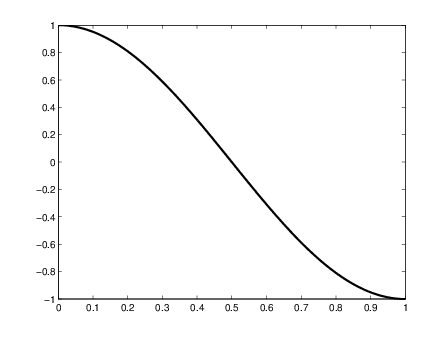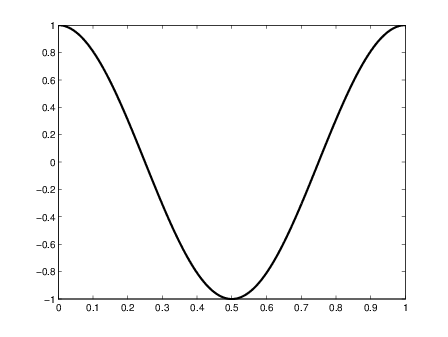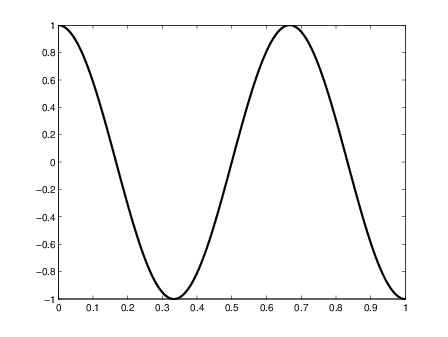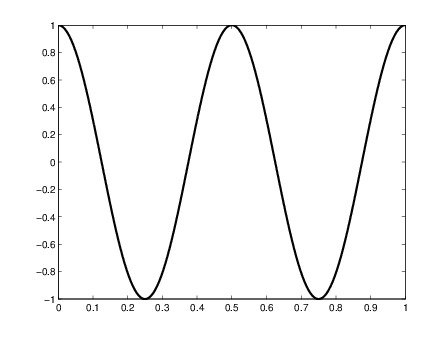Univariate Fourier basis functions for $j = 1, 2, 3$ and $4$. The basis function for $j = 0$ is a constant.

For multivariate state spaces, where s ∈ ℜd$\left(where each elements$i of s is scaled to $\left[0, 1\right]$) the $n$th order Fourier basis is defined by the set of basis functions:

$\phi$j(s) = cos(π cj · s),

where each cj is a length $d$coefficient vector, each element of which is an integer between $0$ and $n$. The basis is obtained by enumerating all such coefficient vectors.

A few example basis functions for a 2D domain are shown below. Each element of the cj vector specifies the basis function's frequency along the corresponding state variable; for example, in a 2D domain, cj = [1, 2] results in a basis function with frequency $1$ along $s$1 and frequency $2$ along $s$2. Setting a state variable's coefficient to zero results in a basis function that is invariant to changes along that state dimension.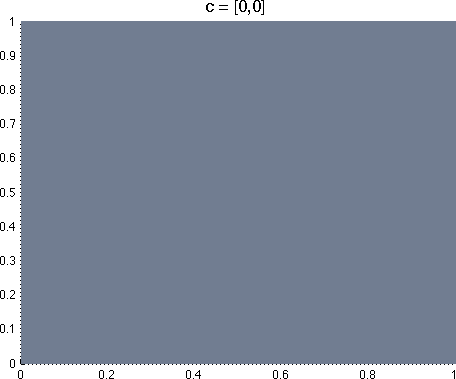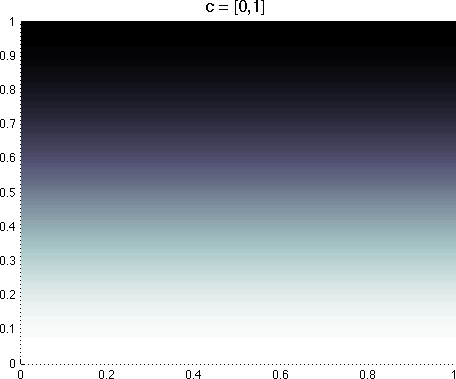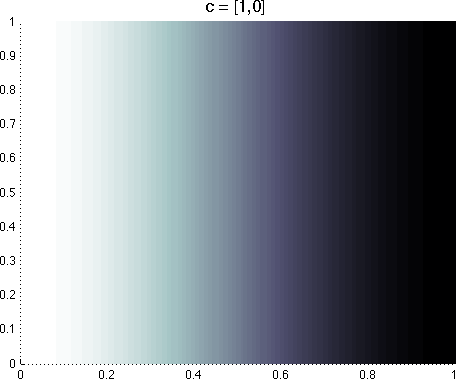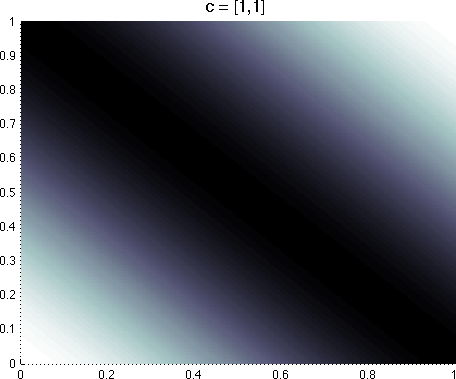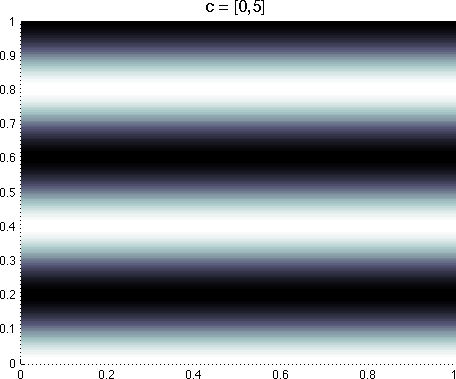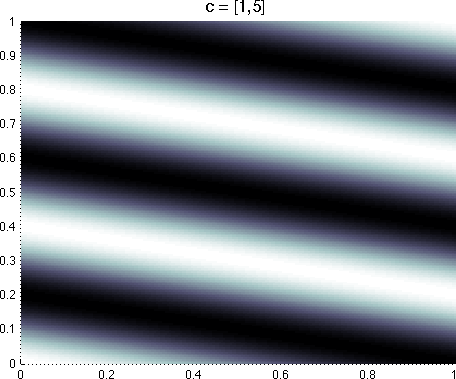A few example Fourier basis functions defined over two state variables. Lighter colors indicate a value closer to $1$, darker colors indicate a value closer to $-1$.

One caveat: when performing gradient-descent style value function approximation (such as TD($\lambda$)), we must deal with the fact that some Fourier basis functions have much higher frequencies than others, and scale their gradient descent step sizes appropriately. In practice, we've found that setting $\alpha$i = α0/||ci||2, where $\alpha$0 is the base learning rate assigned to the basis function with c0, performs reliably well.

An $n$th order Fourier basis in a $d$-dimensional space has $\left(n + 1\right)d$ basis functions, and thus suffers the combinatorial explosion in $d$ exhibited by all complete fixed basis methods. In a domain where $d$ is sufficiently small - perhaps less than 6 or 7 - we may simply pick an order $n$ and enumerate all basis functions. This amounts to an expectation that components of the value function with frequency $n$ or less are signal, and components with higher frequency are noise. For higher dimensional domains, we may reduce the size of the basis by placing restrictions on the cj vectors. For example, we can include only coefficient vectors with two or fewer non-zero elements; this is analogous to tile coding pairs of variables.

The paper describing the Fourier Basis is:

Source Code:

Here is a list of papers using the Fourier basis, courtesy of Google Scholar.